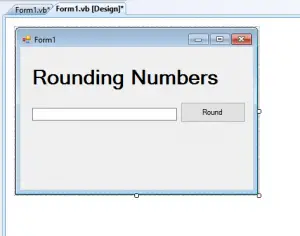# How to Round a Number in VB.Net

This Tutorial is all about How to Round a Number in VB.Net. In this tutorial about How to Round a Number in VB.Net, you will be able to Round a Number in VB.Net. So lets get Started:

The most well-known kind of rounding is away from zero, although in financial and statistical processes, rounding to the closest even is the norm. It complies with part 4 of IEEE Standard 754. Rounding to closest decreases the rounding error generated by consistently rounding midpoint values in a single direction when applied in repeated rounding operations. This rounding error can be considerable in some instances.

The bias that might emerge from continuously rounding midpoint values in a single direction is illustrated in the following example. The example calculates the true mean of an array of Decimal values, as well as the mean when the array’s values are rounded using the two conventions. In this case, the true mean and the mean obtained by rounding to the nearest integer are the same. The mean that follows from rounding away from zero, on the other hand, differs by.05 (or by 3.6 percent )

• First is open the Visual Basic, Select File on the menu, then click New and create a new project.
• Then a New Project Dialog will appear. You can rename your project, depending on what you like to name it. After that click OK

• After that, design your form like this just like what I’ve shown you below
Add a Button and a Textbox.• Then Add this Following codes in the Button.

[vbnet]

Private Sub Button1_Click(ByVal sender As System.Object, ByVal e As System.EventArgs) Handles Button1.Click
Dim a As Single
Dim b As Double
b = Convert.ToDouble(TextBox1.Text)
a = Math.Round(b)

TextBox1.Text = a
End Sub
[/vbnet]

• Finally, Click F5 to run the program.

If you have any comments or suggestions about on How to Round a Number in VB.Net, please feel free to contact our webpage.